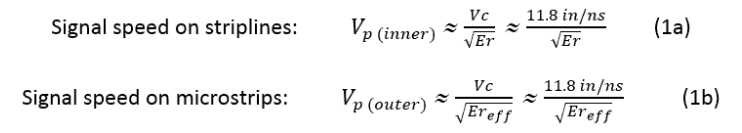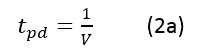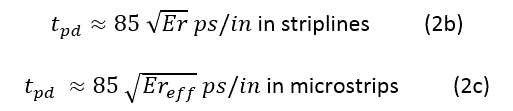# PCB Design How to Calculate Trace Length from Time Delay Value for High-speed Signals

To keep a good high-speed signal quality from driver to receiver on a PCB is not an easy task for designers. One of the most challenging issues is managing the propagation delay and relative time delay mismatches. To manage the time delays in PCB design, we need to know how to calculate trace length from time delay value in order to implement the PCB trace routing accordingly. Let me take you through the process…

### Calculating signal speed on a PCB

According to physics, electromagnetic signals travel in a vacuum or through the air at the same speed as light, which is:

Vc = 3 x 108M/sec = 186,000 miles/second = 11.8 inch/nanosecond

A signal travels on a PCB transmission line at a slower speed, affected by the dielectric constant (Er) of the PCB material. The transmission line structure also affects the signal speed.

There are two general PCB trace structures [note*]: stripline and microstrip.

The formulas for calculating the signal speed on a PCB are given below:Where:

• Vcis the velocity of light in a vacuum or through the air
• Er is the dielectric constant of the PCB material
• Ereffis the effective dielectric constant for microstrips; its value lies between 1 and Er, and is approximately given by:

Ereff≈(0.64 Er+ 0.36)     (1c)

With those formulas, we know that the speed of signals on a PCB is less than the signal speed through the air. If Er≈4 (like for FR4 material types), then the speed of signals on a stripline is half that of the speed through the air, i.e., it is about 6 in/ns.

### How to calculate propagation delay (tpd)

The propagation delay is the time a signal takes to propagate over a unit length of the transmission line.

Here is how we can calculate the propagation delay from the trace length and vice versa:Where:
• Vis the signal speed in the transmission line

In a vacuum or through the air, it equals 85 picoseconds/inch (ps/in).

On PCB transmission lines, the propagation delay is given by:### Case study: Calculating trace length on a PCB

In order to be compliant with the specification of JEDEC, the maximum skew among all the signals shall be less than +/-2.5% of the clock period driven by the memory controller. All the signals of SDRAM are directly or indirectly referenced to the clock.

In this example, the normal FR4 material with a dielectric constant of 4 is used on the PCB with a differential clock rate of 1.2GHz (i.e., 833ps clock period):

Question: What is the maximum skew of the trace length for all the signals?

Answer: Max skew in time delay = +/-2.5% of the 833ps clock period = 20.825ps FR4 Er≈4, Ereff≈2.92

So, for strip lines, the maximum skew should be less than +/- (20.825/(85*SQT(4))=+/-0.1225 in = +/- 122.5 mil.

For microstrips, the maximum skew should be less than +/- (20.825/(85*SQT(2.92)) = +/-0.1433 in = +/- 143.3 mil.

Note*: Different microstrip and stripline structures will affect the signal speed, but only slightly.

Keep this information in mind the next time you’re calculating trace lengths; it should make the job a little easier for you.

References:
–  Signal Speed and Propagation Delay in a PCB Transmission Line, Atar Mittal
–  JEDEC

Also see:

CR-8000 – PCB Design Software Overview

CR-8000 – PCB simulation and analysisLance Wang
Solutions Architect
Lance Wang is a solutions architect at the Zuken SOZO Center. He supports the CR-8000 product line, mainly focusing on high-speed PCB design and signal integrity features. When not behind the keyboard or in front of customers, he is a Tom Brady fan and enjoys playing ping pong in his spare time.